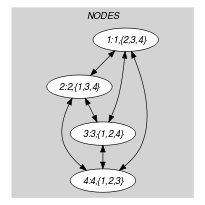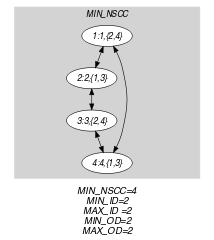## 5.401. tour

Origin
Constraint

$\mathrm{𝚝𝚘𝚞𝚛}\left(\mathrm{𝙽𝙾𝙳𝙴𝚂}\right)$

Synonyms

$\mathrm{𝚊𝚝𝚘𝚞𝚛}$, $\mathrm{𝚌𝚢𝚌𝚕𝚎}$.

Argument
 $\mathrm{𝙽𝙾𝙳𝙴𝚂}$ $\mathrm{𝚌𝚘𝚕𝚕𝚎𝚌𝚝𝚒𝚘𝚗}\left(\mathrm{𝚒𝚗𝚍𝚎𝚡}-\mathrm{𝚒𝚗𝚝},\mathrm{𝚜𝚞𝚌𝚌}-\mathrm{𝚜𝚟𝚊𝚛}\right)$
Restrictions
 $|\mathrm{𝙽𝙾𝙳𝙴𝚂}|\ge 3$ $\mathrm{𝚛𝚎𝚚𝚞𝚒𝚛𝚎𝚍}$$\left(\mathrm{𝙽𝙾𝙳𝙴𝚂},\left[\mathrm{𝚒𝚗𝚍𝚎𝚡},\mathrm{𝚜𝚞𝚌𝚌}\right]\right)$ $\mathrm{𝙽𝙾𝙳𝙴𝚂}.\mathrm{𝚒𝚗𝚍𝚎𝚡}\ge 1$ $\mathrm{𝙽𝙾𝙳𝙴𝚂}.\mathrm{𝚒𝚗𝚍𝚎𝚡}\le |\mathrm{𝙽𝙾𝙳𝙴𝚂}|$ $\mathrm{𝚍𝚒𝚜𝚝𝚒𝚗𝚌𝚝}$$\left(\mathrm{𝙽𝙾𝙳𝙴𝚂},\mathrm{𝚒𝚗𝚍𝚎𝚡}\right)$
Purpose

Enforce to cover an undirected graph $G$ described by the $\mathrm{𝙽𝙾𝙳𝙴𝚂}$ collection with a Hamiltonian cycle.

Example
$\left(\begin{array}{c}〈\begin{array}{cc}\mathrm{𝚒𝚗𝚍𝚎𝚡}-1\hfill & \mathrm{𝚜𝚞𝚌𝚌}-\left\{2,4\right\},\hfill \\ \mathrm{𝚒𝚗𝚍𝚎𝚡}-2\hfill & \mathrm{𝚜𝚞𝚌𝚌}-\left\{1,3\right\},\hfill \\ \mathrm{𝚒𝚗𝚍𝚎𝚡}-3\hfill & \mathrm{𝚜𝚞𝚌𝚌}-\left\{2,4\right\},\hfill \\ \mathrm{𝚒𝚗𝚍𝚎𝚡}-4\hfill & \mathrm{𝚜𝚞𝚌𝚌}-\left\{1,3\right\}\hfill \end{array}〉\hfill \end{array}\right)$

The $\mathrm{𝚝𝚘𝚞𝚛}$ constraint holds since its $\mathrm{𝙽𝙾𝙳𝙴𝚂}$ argument depicts the following Hamiltonian cycle visiting successively the vertices 1, 2, 3 and 4.

Symmetry

Items of $\mathrm{𝙽𝙾𝙳𝙴𝚂}$ are permutable.

Algorithm

When the number of vertices is odd (i.e., $|\mathrm{𝙽𝙾𝙳𝙴𝚂}|$ is odd) a necessary condition is that the graph is not bipartite. Other necessary conditions for filtering the $\mathrm{𝚝𝚘𝚞𝚛}$ constraint are given in [Cymer13], [CymerPhD13].

Keywords
Arc input(s)

$\mathrm{𝙽𝙾𝙳𝙴𝚂}$

Arc generator
$\mathrm{𝐶𝐿𝐼𝑄𝑈𝐸}$$\left(\ne \right)↦\mathrm{𝚌𝚘𝚕𝚕𝚎𝚌𝚝𝚒𝚘𝚗}\left(\mathrm{𝚗𝚘𝚍𝚎𝚜}\mathtt{1},\mathrm{𝚗𝚘𝚍𝚎𝚜}\mathtt{2}\right)$

Arc arity
Arc constraint(s)
$\begin{array}{c}\mathrm{𝚒𝚗}_\mathrm{𝚜𝚎𝚝}\left(\mathrm{𝚗𝚘𝚍𝚎𝚜}\mathtt{2}.\mathrm{𝚒𝚗𝚍𝚎𝚡},\mathrm{𝚗𝚘𝚍𝚎𝚜}\mathtt{1}.\mathrm{𝚜𝚞𝚌𝚌}\right)⇔\hfill \\ \mathrm{𝚒𝚗}_\mathrm{𝚜𝚎𝚝}\left(\mathrm{𝚗𝚘𝚍𝚎𝚜}\mathtt{1}.\mathrm{𝚒𝚗𝚍𝚎𝚡},\mathrm{𝚗𝚘𝚍𝚎𝚜}\mathtt{2}.\mathrm{𝚜𝚞𝚌𝚌}\right)\hfill \end{array}$
Graph property(ies)
$\mathrm{𝐍𝐀𝐑𝐂}$$=|\mathrm{𝙽𝙾𝙳𝙴𝚂}|*|\mathrm{𝙽𝙾𝙳𝙴𝚂}|-|\mathrm{𝙽𝙾𝙳𝙴𝚂}|$

Arc input(s)

$\mathrm{𝙽𝙾𝙳𝙴𝚂}$

Arc generator
$\mathrm{𝐶𝐿𝐼𝑄𝑈𝐸}$$\left(\ne \right)↦\mathrm{𝚌𝚘𝚕𝚕𝚎𝚌𝚝𝚒𝚘𝚗}\left(\mathrm{𝚗𝚘𝚍𝚎𝚜}\mathtt{1},\mathrm{𝚗𝚘𝚍𝚎𝚜}\mathtt{2}\right)$

Arc arity
Arc constraint(s)
$\mathrm{𝚒𝚗}_\mathrm{𝚜𝚎𝚝}$$\left(\mathrm{𝚗𝚘𝚍𝚎𝚜}\mathtt{2}.\mathrm{𝚒𝚗𝚍𝚎𝚡},\mathrm{𝚗𝚘𝚍𝚎𝚜}\mathtt{1}.\mathrm{𝚜𝚞𝚌𝚌}\right)$
Graph property(ies)
 $•$$\mathrm{𝐌𝐈𝐍}_\mathrm{𝐍𝐒𝐂𝐂}$$=|\mathrm{𝙽𝙾𝙳𝙴𝚂}|$ $•$$\mathrm{𝐌𝐈𝐍}_\mathrm{𝐈𝐃}$$=2$ $•$$\mathrm{𝐌𝐀𝐗}_\mathrm{𝐈𝐃}$$=2$ $•$$\mathrm{𝐌𝐈𝐍}_\mathrm{𝐎𝐃}$$=2$ $•$$\mathrm{𝐌𝐀𝐗}_\mathrm{𝐎𝐃}$$=2$

Graph model

The first graph property enforces the subsequent condition: If we have an arc from the ${i}^{th}$ vertex to the ${j}^{th}$ vertex then we have also an arc from the ${j}^{th}$ vertex to the ${i}^{th}$ vertex. The second graph property enforces the following constraints:

• We have one strongly connected component containing $|\mathrm{𝙽𝙾𝙳𝙴𝚂}|$ vertices,

• Each vertex has exactly two predecessors and two successors.

Part (A) of Figure 5.401.1 shows the initial graph from which we start. It is derived from the set associated with each vertex. Each set describes the potential values of the $\mathrm{𝚜𝚞𝚌𝚌}$ attribute of a given vertex. Part (B) of Figure 5.401.1 gives the final graph associated with the Example slot. The $\mathrm{𝚝𝚘𝚞𝚛}$ constraint holds since the final graph corresponds to a Hamiltonian cycle.

##### Figure 5.401.1. Initial and final graph of the $\mathrm{𝚝𝚘𝚞𝚛}$ set constraint(a) (b)
Signature

Since the maximum number of vertices of the final graph is equal to $|\mathrm{𝙽𝙾𝙳𝙴𝚂}|$, we can rewrite the graph property $\mathrm{𝐌𝐈𝐍}_\mathrm{𝐍𝐒𝐂𝐂}=|\mathrm{𝙽𝙾𝙳𝙴𝚂}|$ to $\mathrm{𝐌𝐈𝐍}_\mathrm{𝐍𝐒𝐂𝐂}\ge |\mathrm{𝙽𝙾𝙳𝙴𝚂}|$ and simplify $\underline{\overline{\mathrm{𝐌𝐈𝐍}_\mathrm{𝐍𝐒𝐂𝐂}}}$ to $\overline{\mathrm{𝐌𝐈𝐍}_\mathrm{𝐍𝐒𝐂𝐂}}$.